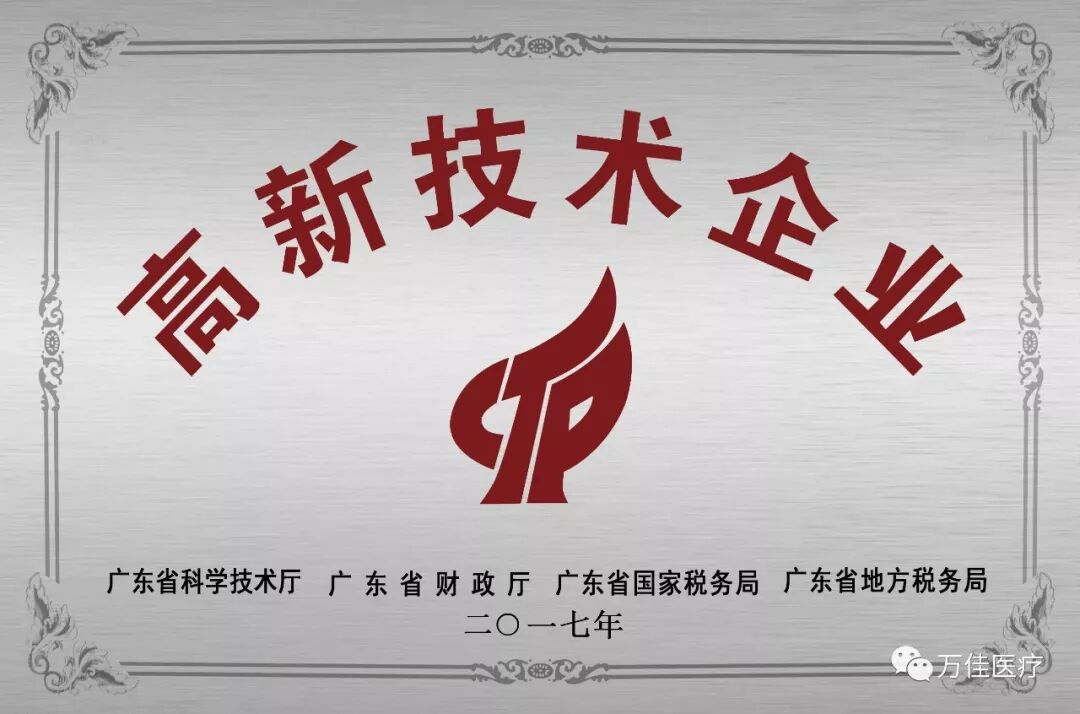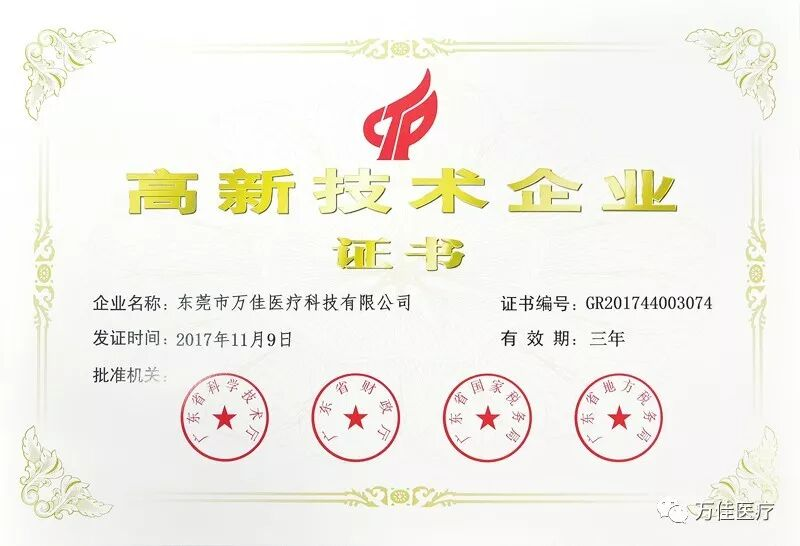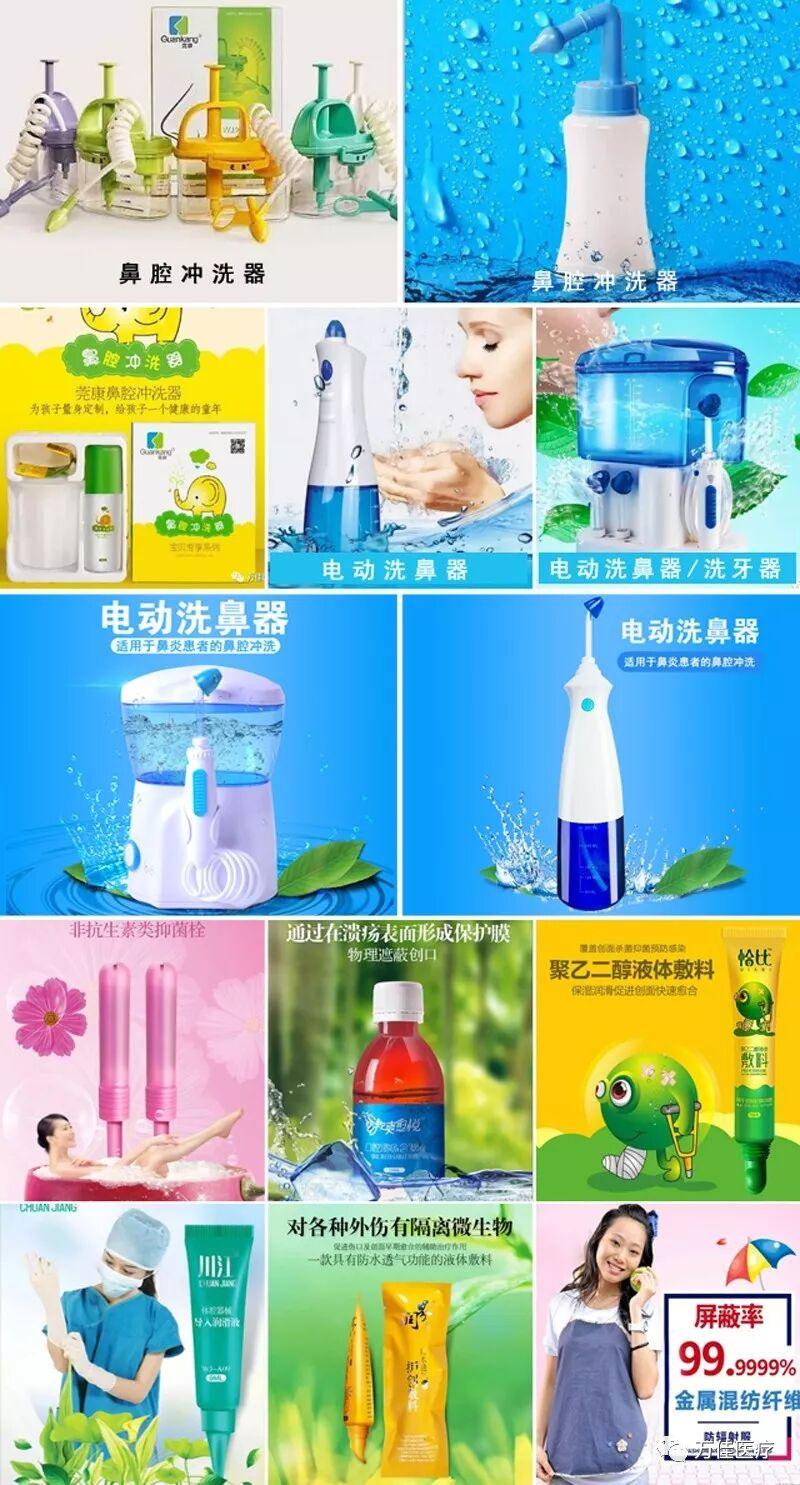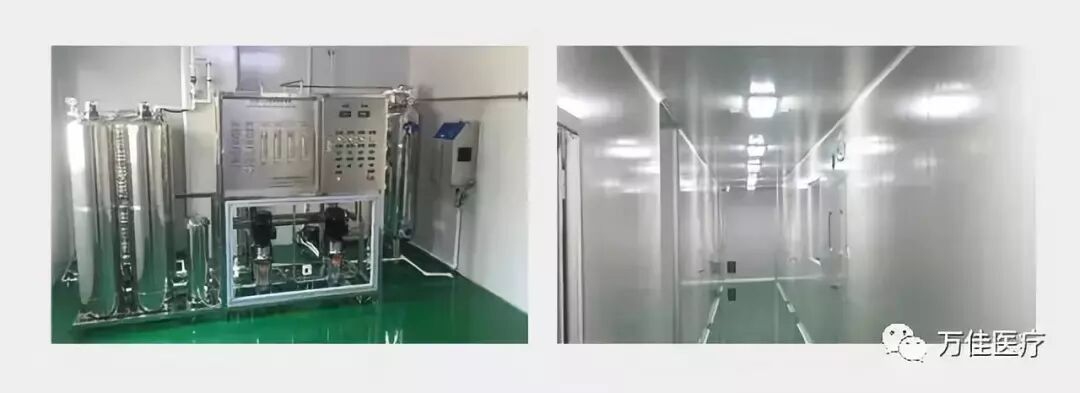医疗器械认证企业  /  OEM生产企业  /  全国免费服务热线 : 400-889-20132018-05-18

特大喜讯！怡越医疗(广东)有限公司荣获国家级高新技术企业资格

喜讯！喜讯！怡越医疗(广东)有限公司(以下简称：怡越医疗)通过国家级高新技术企业认定，喜获牌匾和证书。近日，公司收到了由广东省科学技术厅、广东省财政厅、广东省国家税务局、广东省地方税务局联合颁发的《高新技术企业证书》，发证时间为2017年11月9日，有效期三年。怡越医疗一直致力于生产高品质高性能产品。2012年至今，公司主营线下品牌“莞康、怡越、漱爽愉悦、怡越、川江”等，主要产品有：鼻腔冲洗器、电动洗鼻器、防水透气护创敷料、聚乙二醇液体敷料、口腔溃疡含漱液、活性炭阴道填塞、体腔器械导入润滑液等。在全国各大医院药房、药店均有销售。在电子商务蓬勃发展的今天，公司也不甘落后。除了做好自己的线下品牌，近两年来，我们精心研发生产的“洗鼻器系列产品”，经广东省食品药品监督管理局审核通过批准生产，因为品质保证，各证齐全，不断得到网商的青睐，天猫商城医疗器械类的销售商都一直跟我们保持最密切的合作，而且销量在同类产品中也相当不错。我们酝酿已久的电商品牌——“见善”也应运而生。“见善”品牌取意于“见贤思齐，尽善尽美”之义。目前，见善牌洗鼻器系列产品已在京东和淘宝正常运营，发展态势良好。

关于怡越

<<<<<<<<<<<<<<<<<<<<<<<<<<<<<<<<<<<<<<<<

<<<<<<<<<<<<<<<<<<<<<<<<<<<<<<<<<<<<<<<<

“十万级洁净厂房500平方米”

<<<<<<<<<<<<<<<<<<<<<<<<<<<<<<<<<<<<<<<<<<<<<<<<<<<<<<<<<<<<<<<<<<<<<<<<<<<<<<<<

“二类医疗器械批准文号8个”

“一类医疗器械批准文号1个”

<<<<<<<<<<<<<<<<<<<<<<<<<<<<<<<<<<<<<<<<« 上一篇  下一篇 »
关注我们官方网站微信公众号